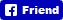﻿ Fun Easy English - new american english language vocabulary pictures words dictionary study practice flashcards presentation kids mathematics subtraction zero and positive integers.
 Classroom Pronunciation Reductions Grammar Videos Conversation Reading Activities Listening Study Idioms Slang Acronyms Surveys Tests Alphabet Alphabet 4 Kids Vocabulary Travel America Drive AmericaFollow @FunEasyEnglish
 Subtraction Zero and Positive Integers
 Flashcards 4 Kids In this lesson you will use flashcards to improve your English. Read the flashcards description, instructions, information, and do the flashcards.
 Flashcards 4 Kids - Subtraction Zero and Positive Integers
 Flashcards 4 Kids - Subtraction Zero and Positive Integers Description This lesson has mathematics flashcards with descriptions in English. Flashcards 4 Kids - Subtraction Zero and Positive Integers Instructions Press the PLAY button to begin the flashcard presentation each slide shows for 3 seconds Press the FORWARD button to advance one slide ahead with no time limit Press the BACK button to advance one slide back with no time limit Use a dictionary if you cannot understand a word
 Flashcards 4 Kids - Subtraction Zero and Positive Integers Information This set of Fun Easy English flashcards contains the following calculations along with their descriptions: 1 - 1 = 0 2 - 1 = 1 2 - 2 = 0 3 - 1 = 2 3 - 2 = 1 3 - 3 = 0 4 - 1 = 3 4 - 2 = 2 4 - 3 = 1 4 - 4 = 0 5 - 1 = 4 5 - 2 = 3 5 - 3 = 2 5 - 4 = 1 5 - 5 = 0 6 - 1 = 5 6 - 2 = 4 6 - 3 = 3 6 - 4 = 2 6 - 5 = 1 6 - 6 = 0 7 - 1 = 6 7 - 2 = 5 7 - 3 = 4 7 - 4 = 3 7 - 5 = 2 7 - 6 = 1 7 - 7 = 0 8 - 1 = 7 8 - 2 = 6 8 - 3 = 5 8 - 4 = 4 8 - 5 = 3 8 - 6 = 2 8 - 7 = 1 8 - 8 = 0 9 - 1 = 8 9 - 2 = 7 9 - 3 = 6 9 - 4 = 5 9 - 5 = 4 9 - 6 = 3 9 - 7 = 2 9 - 8 = 1 9 - 9 = 0 10 - 1 = 9 10 - 2 = 8 10 - 3 = 7 10 - 4 = 6 10 - 5 = 5 10 - 6 = 4 10 - 7 = 3 10 - 8 = 2 10 - 9 = 1 10 - 10 = 0 Please post a comment below if these flashcards are no longer working. Add Flashcards 4 Kids - Subtraction Zero and Positive Integers to YOUR page, blog, website Simply cut and paste the following: Send Flashcards 4 Kids - Subtraction Zero and Positive Integers to YOUR friends Simply cut and paste the following: https://docs.google.com/presentation/pub?id=14oDJ34hJRS-yEyUseZ-48oYq-vqUIVr-z-Wx6KMHdfc&start=false&loop=false&delayms=3000Follow @FunEasyEnglish